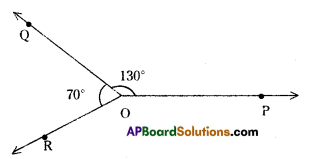AP State Syllabus AP Board 7th Class Maths Solutions Chapter 4 Lines and Angles InText Questions and Answers.

## AP State Syllabus 7th Class Maths Solutions 4th Lesson Lines and Angles InText QuestionsTry This

Question 1.
How many transversals can be drawn for two distinct lines? (Page No.77)
Solution:
Infinite number of transversals can be drawn for two distinct lines.

DoThis:

Question 1.
Identify the transversal in figure (1) and (ii). Identify the exterior and interior angles and fill the table given below. (Page No. 78)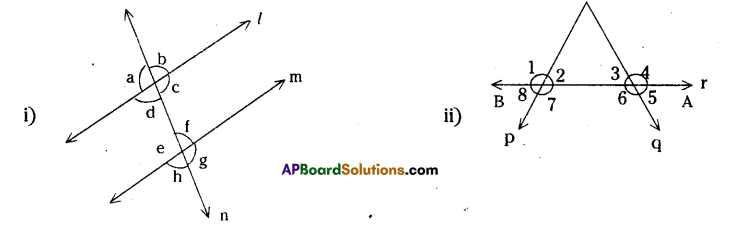Solution:

 Figure Transversal Exterior angles Interior angles i) n ∠a, ∠b, ∠g, ∠h ∠c, ∠d, ∠e, ∠f ii) r ∠1, ∠4, ∠5, ∠8 ∠2, ∠3, ∠6, ∠7Question 2.
Consider the following lines. Which line ¡s a transversal. Number and list all the angles formed. Which are the exterior angles and which are the interior angles (Page No.79)
Solution: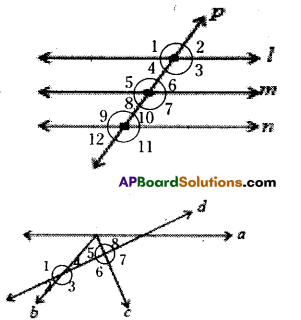For fig. (i) P is transversal for l, m and n.
∠3, ∠4, ∠5, ∠6 are interior angles for l, m.
∠7, ∠8, ∠9, ∠10 are Interior angles for m, n.
∠1, ∠2, ∠7, ∠8 are exterior angles for l, m.
∠1, ∠2, ∠11, ∠12 are exterior angles for l, n.
for fig (ii),
line d is transversal for pair of lines b and c.
∠3, ∠4, ∠5, ∠6 are interior angles.
∠1, ∠2, ∠7, ∠8 are exterior angles.

Do This

Question 1.
Name the pairs of angles in each figure by their property. (Page no. 80)Question 2.
Fill the table with the measures of the corresponding angles. (Page no. 83)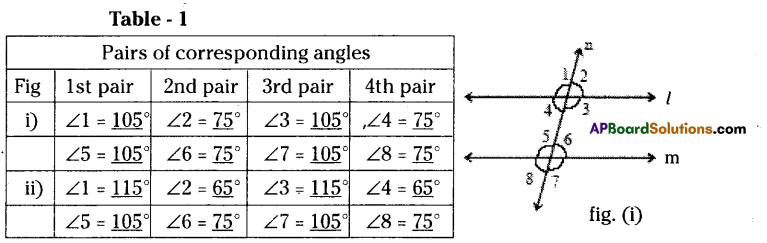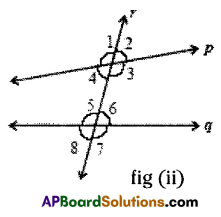1. Find out in which figure the pairs of corresponding angles are equal
In fig. (I) pairs of corresponding angles are equal. q

2. What can you say about the lines ‘1’ and ‘m’?
l is parallel to m, fig (ii)

3. What can you say about the lines ‘p’ and ‘q’?
In fig. (ii) pairs of corresponding angles are not equal and hence p is not parallel to q.

4. Which pair of lines are parallel?
L and M are parallel.

Fill the table with the measures of the interior alternate angles. (Page no. 84)

Table – 2

 Fig Pairs of interior alternate angles 1st pair 2nd pair i) ∠3 = 105° ∠4 = 75° ∠5 = 105° ∠6 = 75° ii) ∠3 = 115° ∠4 = 65° ∠5 = 105° ∠6 = 75°

1. Find out in which the pair of interior ¿ ternate angles are equal?
In fig (i) the pairs of alternate interior angles are equal.

2. What can you say about lines ‘L’ and ‘M’?
L||m.

3. What can you say about the lines ‘p’ and ‘q’?
In fig (ii) the pair of alternate interior angles are not equal. Therefore p ∦ q

Try This

Question 1.
Write any five pairs of supplementary angles of your choice. (Page no 72)
Solution:
(80°, 100°), (60°, 120°), (108°, 72°) (140°, 40°). 30°, 150°)Try This

Question 1.
Draw five pairs of complementary angles of your choice. (Page no. 71)
Solution:
The following are the pairs of complementary angles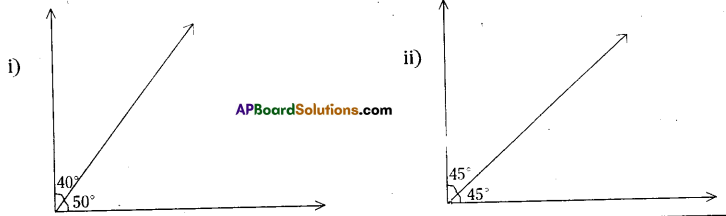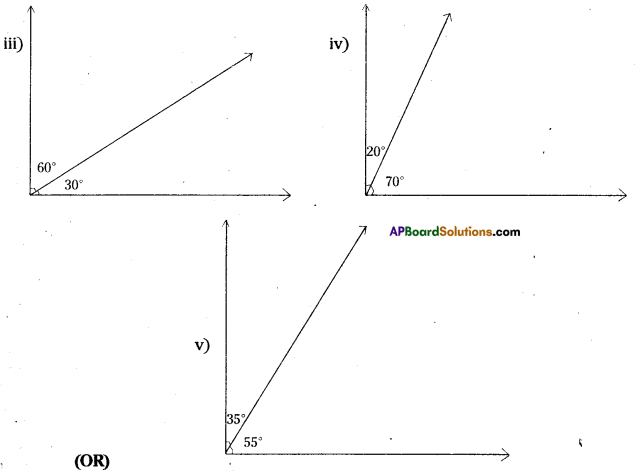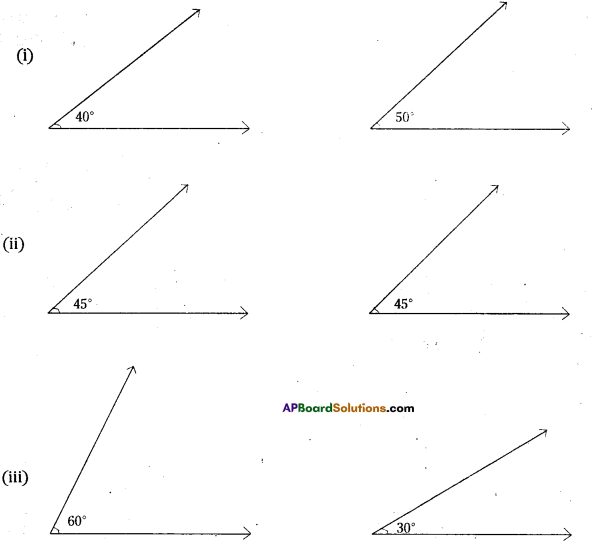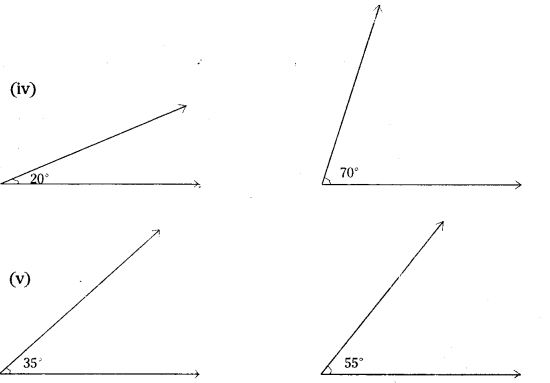Do This

Question 1.
Draw an angle ∠AOB = 40°. With the same vertex 0’ draw ∠BOC = 50°, taking $$\overrightarrow{\mathbf{O B}}$$ Initial ray as shown In the figure. Since the sum of these angles Is 90°, they together form a right angle. Take another pair 60° and 50° and join in the same way. Do they form complementary angles? Why? Why not? (Page No. 71)
Solution:
∠AOB = 40°, ∠BOC = 50°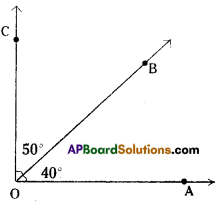∠POQ = 60°
∠QOR = 50°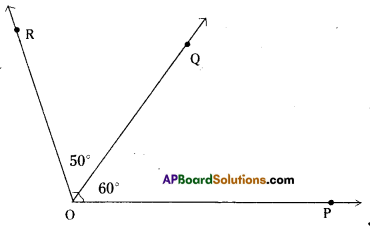These two do not form pair of complementary angles since their sum is 60° + 50° = 110° ≠ 90°

Question 2.
Draw an angle ∠AOB = 100° with the same vertex O, draw ∠BOC = 80° such that $$\overline{\mathrm{OB}}$$ is common to two angles. (Page No. 72)
Solution:
∠AOB = 100°
∠BOC = 80°
∠AOB +∠BOC = 180°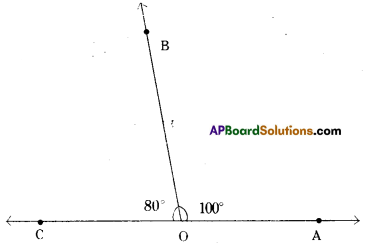Question 3.
Are 130° and 70° supplementary angles? Why? Why not?
∠POQ = 130°, ∠QOR = 70°
∠POQ + ∠QOR = 130° + 70° = 200° ≠ 180°
Hence they are not supplementary angles.